How Cheenta works to ensure student success?
Explore the Back-Story

# TIFR 2014 Problem 29 Solution - Maps from compact spacesTIFR 2014 Problem 29 Solution is a part of TIFR entrance preparation series. The Tata Institute of Fundamental Research is India's premier institution for advanced research in Mathematics. The Institute runs a graduate programme leading to the award of Ph.D., Integrated M.Sc.-Ph.D. as well as M.Sc. degree in certain subjects.
The image is a front cover of a book named Introduction to Real Analysis by R.G. Bartle, D.R. Sherbert. This book is very useful for the preparation of TIFR Entrance.

Also Visit: College Mathematics Program of Cheenta

## Problem:

Let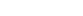be a continuous map between metric spaces. Then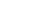is a complete subset of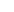if

A. X is compact

B. Y is compact

C. X is complete

D. Y is complete

Discussion:

Let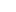be compact. Thenis compact. (continuous image of compact space is compact)

Now, compact subset of any Hausdorff space is closed. So in particular, compact subset of any metric space is closed.

Let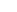be a Cauchy sequence in. Then sinceis compact,has a convergent subsequence (converging to a point in that compact set i.e, in.

Suppose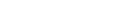.

Then by triangle inequality, we have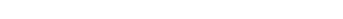as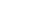Here we have used thatis cauchy to conclude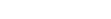.

So this implies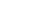. Since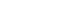we conclude thatis complete.

This proves A.

Let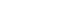and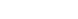. Take the inclusion map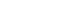for all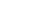. This example shows that even if we taketo be compact, or complete,need not be complete. So this disproves B and D.

Now take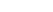and take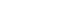. We know there is a homeomorphism between these two sets where the metric is usual topology. So, in this case, the image of a complete set is not complete. This disproves option C.

## Helpdesk

• What is this topic: Real Analysis
• What are some of the associated concept: Cauchy Sequence,Homeomorphishm, Compact Space
• Book Suggestions: Introduction to Real Analysis by R.G. Bartle, D.R. SherbertTIFR 2014 Problem 29 Solution is a part of TIFR entrance preparation series. The Tata Institute of Fundamental Research is India's premier institution for advanced research in Mathematics. The Institute runs a graduate programme leading to the award of Ph.D., Integrated M.Sc.-Ph.D. as well as M.Sc. degree in certain subjects.
The image is a front cover of a book named Introduction to Real Analysis by R.G. Bartle, D.R. Sherbert. This book is very useful for the preparation of TIFR Entrance.

Also Visit: College Mathematics Program of Cheenta

## Problem:

Letbe a continuous map between metric spaces. Thenis a complete subset ofif

A. X is compact

B. Y is compact

C. X is complete

D. Y is complete

Discussion:

Letbe compact. Thenis compact. (continuous image of compact space is compact)

Now, compact subset of any Hausdorff space is closed. So in particular, compact subset of any metric space is closed.

Letbe a Cauchy sequence in. Then sinceis compact,has a convergent subsequence (converging to a point in that compact set i.e, in.

Suppose.

Then by triangle inequality, we haveasHere we have used thatis cauchy to conclude.

So this implies. Sincewe conclude thatis complete.

This proves A.

Letand. Take the inclusion mapfor all. This example shows that even if we taketo be compact, or complete,need not be complete. So this disproves B and D.

Now takeand take. We know there is a homeomorphism between these two sets where the metric is usual topology. So, in this case, the image of a complete set is not complete. This disproves option C.

## Helpdesk

• What is this topic: Real Analysis
• What are some of the associated concept: Cauchy Sequence,Homeomorphishm, Compact Space
• Book Suggestions: Introduction to Real Analysis by R.G. Bartle, D.R. Sherbert

This site uses Akismet to reduce spam. Learn how your comment data is processed.

### Knowledge Partner